International Journal of Energy and Power Engineering
Volume 4, Issue 2, April 2015, Pages: 84-93

Dynamic Economic Dispatch for Combined Heat and Power Units using Particle Swarm Algorithms

Mohamed Ahmed Sadeek1, Azza Ahmed El Dessouky2, Abd El Hay Ahmed Sallam2

1East Delta Electricity Production Company, Ismailia, Egypt

2Faculty of engineering, Port-Said University, Port-Said, Egypt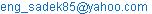(M. A. Sadeek)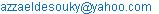(A. A. E. Desouky)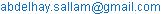(A. E. H. A. Sallam)

Mohamed Ahmed Sadeek, Azza Ahmed El Dessouky, Abd El Hay Ahmed Sallam. Dynamic Economic Dispatch for Combined Heat and Power Units using Particle Swarm Algorithms. International Journal of Energy and Power Engineering. Vol. 4, No. 2, 2015, pp. 84-93. doi: 10.11648/j.ijepe.20150402.19

Abstract: In this paper, combined heat and power units are incorporated in dynamic economic dispatch to minimize total production costs considering realistic constraints such as ramp rate and spinning reserve limits effects over a short time span. Four evolutionary approaches, namely particle swarm optimization (PSO), particle swarm optimization with constriction factor (PSOCFA), particle swarm optimization with inertia weight factor (PSOIWA) and particle swarm optimization with both constriction factor and inertia weight factor (PSOCFIWA) are successfully implemented to solve the combined heat and power economic dispatch (CHPED) problem. These approaches have been tested on 12-generation units system with two steam, four gas and six cogeneration units. In addition, the performance tests are applied to measure the actual power output and the fuel consumption in every point tests for achieving different curves such as input/output, incremental heat rate and heat rate curves for the twelve units. The results of the four approaches are compared with those obtained using existing performance testing method. The results show that the particle swarm optimization with improved inertia weight is able to achieve a better solution at less computational time.

Keywords: Combined Heat and Power Economic Dispatch (CHPED), Spinning Reserve, Ramp Rate, Particle Swarm Optimization (PSO)

1. Introduction

Combined heat and power unit (CHPU) known as cogeneration has the ability of creating simultaneous generation of two types of energy: useful heat and electricity. It improves efficiency and therefore, is more environmental friendly . It also reduces the generation cost between 10 and 40% . In Thermal Units, all the thermal energy is not converted into electricity and large quantities of energy are wasted in the form of heat . CHPU uses the heat and can potentially achieve the energy conversion efficiency of up to 80% . This means that less fuel needs to be consumed to produce the same amount of useful energy.

In order to utilize the CHPUs more efficiently, economic dispatch must be applied to achieve their optimal combination of power and heat output subject to system equality and inequality operational constraints. Hence, the combined heat and power economic dispatch (CHPED) problem is formulated as an optimization problem . A practical CHPED problem should include ramp rate limits, spinning reserve to overcome the sudden fault in the system and joint characteristic of electricity power heat which makes finding the optimal dispatching a challenging problem[6,7].

In the recent researches, global optimization techniques like genetic algorithms (GA) , harmony search algorithm (HAS) , and particle swarm optimization (PSO) , have been applied for optimal tuning of CHPED based restructure schemes. These evolutionary algorithms are heuristic population-based search procedures that incorporate random variation and selection operators. Although, these methods seem to be good methods for the solution of CHPED parameter optimization problem, they have degraded efficiency to obtain global optimum solution when the system has a highly epistatic objective function (i.e. where parameters being optimized are highly correlated), and number of parameters to be optimized are large, then. In order to overcome these drawbacks, different modifications of particle swarm optimization approach are proposed for solution of the CHPED problem [10,11,12].

In this work, heat and power output of each generating unit and optimum fuel cost are obtained by using four approaches; particle swarm optimization (PSO), particle swarm optimization with constriction factor (PSOCFA), particle swarm optimization with inertia weight factor (PSOIWA) and particle swarm optimization with constriction factor and inertia weight factor (PSOCFIWA). The results of the four approaches are compared with those obtained using existing performance testing method. Simulation results show that the PSOIWA approach is superior to the other existing methods.

2. CHPED Problem Formulation

The proposed CHPED problem is an optimization problem like economic load dispatch (ELD) problem, but it considers some types of production units such as pure heat units, cogenerating combined heat and power units. The cogeneration is a role to produce heat and power with feasible operation region according to Figure 1, where the boundary curve ABCDEF determines the feasible region. Along the boundary there is a trade-off between power generation and heat production delivered by the unit. It can be seen that along the curve AB the unit reaches maximum output power. On the contrary, the unit reaches maximum heat production along the curve CD. Therefore, power generation limits of cogeneration units are determined by combined functions incorporating the unit heat production, and vice versa . Mathematically, the problem is formulated as:Figure 1. Typical heat-power region for cogeneration units.

Objective Function:

Minimize:

Cost =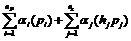(1)

Constraints

Equality constraints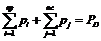(2)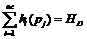(3)

Inequality constraints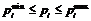, i = 1, ……, np       (4)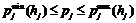, j = 1, …, nc     (5)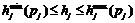, j = 1, ….., nc     (6)

where:

Cost: Total heat and power production cost,

α: Unit production cost,

P: Unit power generation,

h : cogeneration heat production,

HD : System heat demand,

PD : System power demand,

np, nc are the numbers of the of conventional power units and cogeneration units, respectively.

pmin and pmax are the unit power capacity limits,

hmin and hmax are the cogeneration heat capacity limits.

- In addition, up and down ramp rate limits can be formulated as: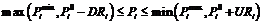(7)

where,

Pi is the output power at time 't', Pio is the initial output power, URi & DRi are the ramp up & down rate limits of the ith generator, respectively.

- Spinning reserve requirements

The Mid American Interconnected Network (MAIN) requires 1.1% of peak demand for regulation. MAIN's additional requirement for spinning reserve is 1.5% of it as peak demand. Thus, the total spinning reserve is allocated among as many units as is practical because it is easier to get the required rapid response by adjusting several units by small amounts rather than by adjusting a single unit by a large amount. The MAIN's non spinning reserve requirement is 1.9 % of the peak demand .

3. Proposed Approaches of PSO

PSO is a population based optimization algorithm . The population is called 'swarm'. Each potential solution is called particle which is given a random velocity and is flown through the solution space searching for the optimal position. Each particle keeps track of its previous best position, called pbest, and corresponding fitness in its memory. The best value of pbest is called gbest, which is the best position discovered by the swarm. If promising new solution is discovered by a particle then all other particles will move closer to it. Based on PSO concept, mathematical equations for the searching process are: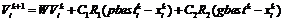(8)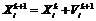(9)

where,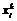,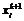are the position of dth dimension (variable) of the ith particle at kth and (k+l)th iteration,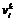,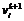are the velocity of the dth dimension of the ith particle at the kth and (k+ l)th iteration. C1, C2 are the cognitive and the social parameters, Rl and R2 are random numbers uniformly distributed within [0, 1], Pbesti is the best position of the dth dimension of the ithparticle, gbesti is the group best position of the dth dimension and w is the inertia weight factor.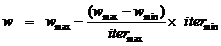(10)

where, itermax is the maximum number of iterations and iter is the current number of iterations.

4. Particle Swarm Optimization with Constriction Factor Approach (PSOCFA)

For particle swarm optimization with constriction factor approach (PSOCFA), the velocity of Equation (8) is manipulated as: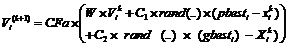(11)

The constriction factor (CFa) varies from 0.60 to 0.73

5. Particle Swarm Optimization with Inertia Weight Factor Approach (PSOIWA)

In inertia weight factor approach (IWA), inertia weight (Wk+1) at (k+1)th cycle is given by :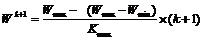(12)

Velocity updating equation: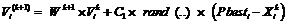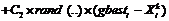(13)

where: Wmax = 1, Wmin = 0.4; Kmax = maximum number of iteration cycle.

6. Particle Swarm Optimization with Constriction Factor & Inertia Weight Factor Approach (PSOCFIWA)

In this approach, the velocity is changed according to the following: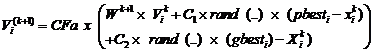(14)

The constriction factor (CFa) varies 0.6 to 0.73 and the inertia weight factor approach (IWA) follows Equation (14).

7. Solution Methodology

The process of the four approaches can be summarized as follows:

Step 1: The particles are randomly generated between the operating limits.

Step 2: The values of the fitness function of the particles are evaluated using objective function, Equation (1) and the dimensions (variables) of the particles are initialized as Pbesti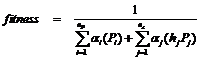Step 3: The best value of pbest(s) is represented as gbest.

Step4: The particles' velocities and positions are updated using velocity and position updating equations corresponding to each approach.

Step 5: The new fitness function values are evaluated using the updated positions of the particles. If the current position of the particle is better than its previous pbest, the pbest is updated by the current particle, otherwise it is not updated. The updated gbest is the best among all the pbest(s).

Step 6: If the stopping criterion is satisfied, go to Step 7, otherwise, go to Step 2.

Step 7: The particle that generates the latest gbest yields the optimal variables. 

8. Performance Tests

Testing and monitoring programs are developed to find out where the efficiency problems are and what improvements can be made. The objective of these performance tests is to provide uniform test methods to obtain the best points of the units operation (optimal power with maximum efficiency). In addition, they help determine the thermal performance and electrical output (capacity or efficiency) of heat cycle for electric power plants and cogeneration facilities according to the specifications [17,18]. Twelve generation units (two steam units of Ayoun Mousa steam power plant, four gas units of West Damietta power plant and six cogeneration units of Damietta combined power plant) with data given in Appendix A are used in this study in order to assess the performance of the four approaches.

In this study, the performance tests are applied to measure the actual power output and the fuel consumption in every point tests to achieve different curves such as input/ output, incremental heat rate and heat rate curves for the 12 units. It has been proved that the intersection of both the hate rate and incremental heat rate curves occurs at the minimum heat rate value. The results of the performance tests for the 12 units are as follow:

A.        Power only units:

- Two steam units

F(Pi) =Limit: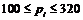and URi = 65 , DRi = 100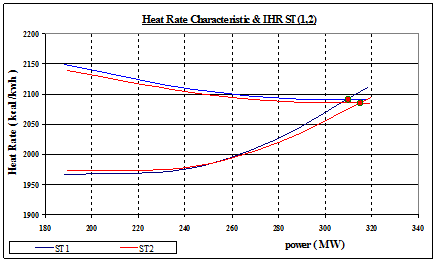Figure 2. Illustrate performance test for 2 steam units.

The fuel costs of the two steam units according to Figure 2 can be expressed as:

- Four gas units: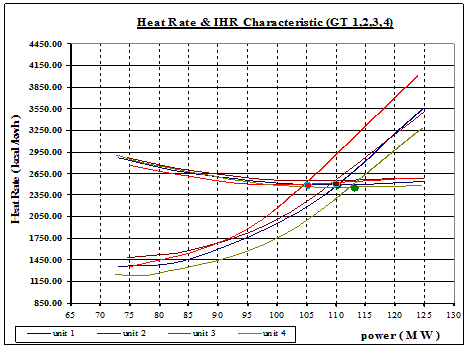Figure 3. Illustrate performance test for 4 gas units.

From Figure 3 the fuel costs of the four gas units can be expressed as:

F(Pi )=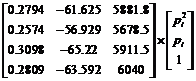Limit: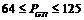, URi = 125 , DRi = 125

where, PGTi is the power limits of gas units.

B.        Cogeneration units: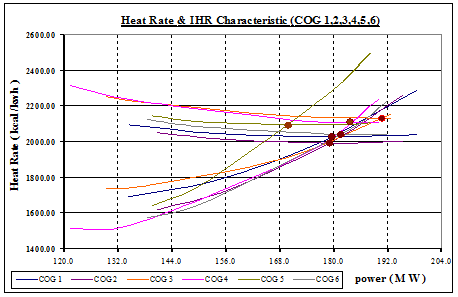Figure 4. Illustrate performance test for 6 cogeneration units.

The combined heat and power cost equation is expressed as follow: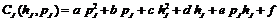where, a, b, c, d, e, and f are the combined heat and power cost equation coefficients and J is the number of cogeneration units.

Figure 4 shows the heat rate and incremental heat rate characteristics for cogeneration units. From this figure, the combined heat and cost is expressed as: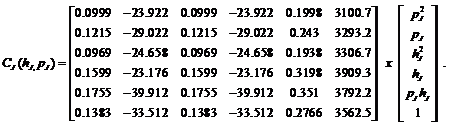Limit: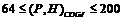,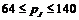,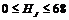, URi = 60 , DRi = 100.

where,

(P,H)COGJ: total power and heat limits of cogeneration units,

PJ: cogeneration power limits and HJ: cogeneration heat limits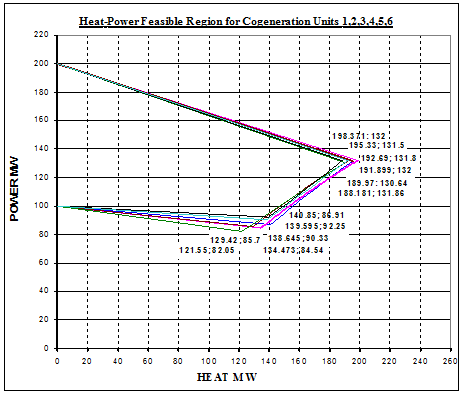Figure 5. The heat-power operating region for 6 cogeneration units.

Figure 5 shows heat-power feasible region for the six cogeneration units. The maximum and minimum fuel is 200 and 100 MW; respectively.

9. Simulation Results

CHPED problem is solved using the PSO, PSOCFA, PSOIWA and PSOCFIWA approaches. To assess the units efficiency when applying each approach, two case-study are proposed. First, the approaches are tested with a load demand equals to 2148 MW which is the reference of the performance test for the twelve generating units. Second, they are applied to a daily load curve. On both cases, twelve units (two steam, four gas and six cogeneration units) are used. For PSO simulation, the population size = 50, and the maximum iteration = 600.

First case study:

Figure 6 shows the convergence behavior of the PSO and other approaches for 12 generating units at load 2148 MW. It is shown that PSOIW approach can reach the best solution with minimum cost. Table 1 shows a comparison between the results of the four approaches with those obtained from the performance test. From these results, it can be seen that the results of PSOIWA approach provides lower total operation cost at less computation time compared with those obtained from the other three approaches. Therefore, PSOIWA is more effective in providing better solutions and shows a more robust performance.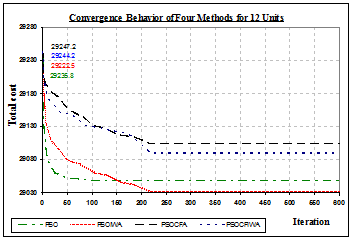Figure 6. The convergence behavior of the PSO and other methods for 12 units at load 2148MW.

Table 1. Comparison results between the PSO, PSOCFA, PSOIWA, and PSOCFIWA approaches with those of performance test.

 Units output PSO PSOIWA PSOCFA PSOCFIWA TESTING ST1 302.391 317.97 286.84 319.98 309 ST2 308.329 306.24 320.00 319.99 319 GA1 110.351 119.49 117.78 78.39 110 GA2 111.673 112.86 103.43 104.41 110 GA3 108.642 84.65 96.64 121.19 105 GA4 82.888 75.26 104.59 112.68 113 COG-P1 133.550 122.430 131.860 130.190 119.92 COG-H1 65.660 60.150 64.730 63.840 59.08 COG-P2 134.910 130.510 117.640 126.900 120.63 COG-H2 62.740 61.220 57.630 60.100 58.37 COG-P3 126.210 134.520 134.060 122.220 129.20 COG-H3 58.960 64.440 64.110 56.730 60.80 COG-P4 119.160 132.500 118.360 109.920 125.87 COG-H4 54.390 59.840 54.060 50.630 57.13 COG-P5 125.430 127.360 140.220 123.300 115.68 COG-H5 56.550 56.980 59.680 56.080 54.32 COG-P6 128.410 124.860 120.770 132.490 124.40 COG-H6 57.750 56.730 55.570 58.950 56.60 Total power (MW) 2148.0 2148.0 2148.0 2148.0 2148.0 Total heat production (MW) 356.05 359.36 355.78 346.33 346.3 Total cost (\$/h) 29049.1 29032.1 29104.4 29090.67 29316.76 CPU Time (sec) 4.65 4.47 4.96 4.87 ---

The total cost of PSOIWA with heat and load demands (\$29032.14) is lower than those of PSO, PSOCFA, PSOCFIWA (\$29049.192, \$29104.45 and \$29090.67, respectively). In addition, the total heat production which is the sum of the total heat production of the six cogeneration units (359.36 MW) is higher than those of the other approaches (356.05 MW, 355.78 MW and 346.33 MW; respectively). The same conclusion can be concluded from Figure 7.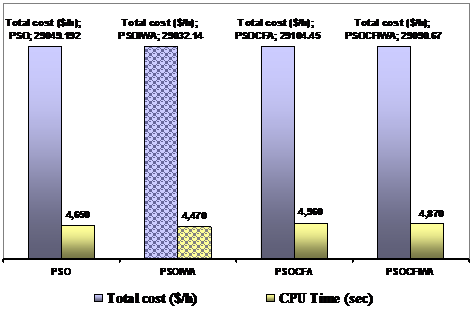Figure 7. The comparison between PSO and other methods for case 1.

Second case study

Figure 8 shows the daily load curve used in the study. The four approaches are applied to the twelve units and Figure 9 shows the comparison between the results. It is evident that the PSOIWA approach has the advantage of cost saving that is around 1.00058, 1.00249 and 1.002016 times from PSO, PSOCFA and PSOCFIWA, respectively.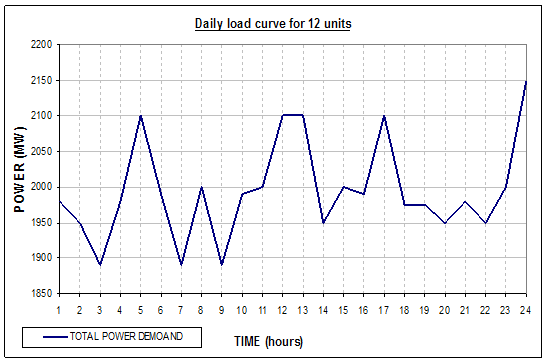Figure 8. the daily load curve.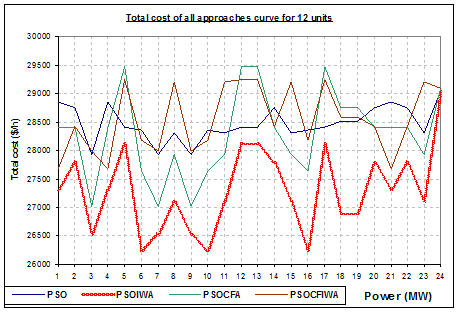Figure 9. The total cost for 12 generation units of all approaches for case 2.

10. Conclusions

Comparative study based on PSO, PSOCFA, PSOIWA and PSOCFIWA approaches applied to solve CHPED problem has been presented. The approaches are tested on 12 generation units (two steam, four gas and six cogeneration units) taking into consideration the system and units constraints. The results of the four approaches are compared with those obtained using existing performance testing method. From the results, it is clear that PSOIWA approach is more effective than other approaches discussed. This gives the best global optimum solution with less computation time than the PSO, PSOCFA and PSOCFIWA techniques.

Appendix A

The system data of twelve units (two steam, four gas and six cogeneration units) are used.

a)         two steam units x 320 MW:

Steam unit 1:

 item unit Test1 Test2 Test3 Test4 Best point IHR K cal /kwh 2110.89 2023.02 1974.08 1966.12 2090.09 Heat rate K cal /kwh 2090.35 2094.18 2110.60 2149.43 2090.09 Power (output) MW 318 277.92 238.32 188.12 309.925

Steam unit 2:

 item unit Test1 Test2 Test3 Test4 Best point IHR K cal /kwh 2094.24 2020.01 1978.29 1973.07 2084.43 Heat rate K cal /kwh 2084.51 2088.75 2104.01 2139.49 2084.44 Power (output) MW 319.34 279.9 240.22 189.58 314.98

b)         four gas units x 125 MW:

Gas unit 1

 item unit Test1 Test2 Test3 Test4 Best point IHR K cal /kwh 3555.20 2235.32 1481.63 1351.32 2483.71 Heat rate K cal /kwh 2542.67 2488.89 2648.49 2872.10 2483.77 Power (output) MW 124.8 106 86 73 110.28

Gas unit 2:

 item unit Test1 Test2 Test3 Test4 Best point IHR K cal /kwh 3511.88 2098.95 1596.01 1482.11 2497.74 Heat rate K cal /kwh 2584.25 2549.73 2687.60 2864.07 2530.85 Power (output) MW 125 102 85.9 74.6 110

Gas unit 3:

 item unit Test1 Test2 Test3 Test4 Best point IHR K cal /kwh 4007.42 2134.01 1597.85 1356.38 2478.83 Heat rate K cal /kwh 2585.40 2489.21 2582.24 2762.63 2478.92 Power (output) MW 123.8 99.5 87 75 105.26

Gas unit 4:

 item unit Test1 Test2 Test3 Test4 Best point IHR K cal /kwh 3275.93 1782.22 1254.95 1248.11 2440.70 Heat rate K cal /kwh 2477.45 2484.05 2759.79 2903.78 2440.91 Power (output) MW 124.6 100.8 79.5 72.6 113.19

c)         six cogeneration units x 200MW:

Cogeneration unit 1:

 item unit Test1 Test2 Test3 Test4 Best point IHR K cal /kwh 2286.44 1983.85 1784.71 1690.33 2026.6 Heat rate K cal /kwh 2038.37 2027.09 2048.76 2094.43 2026.6 Power (output) MW 132 116.95 100.36 84.54 heat(output) MW 198.371 175.904 153.819 134.473 179.595

Cogeneration unit 2:

 item unit Test1 Test2 Test3 Test4 Best point IHR K cal /kwh 2260.04 2015.55 1733.91 1615.87 1993.39 Heat rate K cal /kwh 2004.00 1993.48 2012.81 2052.78 1993.39 Power (output) MW 131.5 120.6 101.34 86.91 heat(output) MW 195.33 180.66 157.25 140.85 179.148

Cogeneration unit 3:

 item unit Test1 Test2 Test3 Test4 Best point IHR K cal /kwh 2153.21 1910.48 1746.90 1738.49 2130.2 Heat rate K cal /kwh 2130.31 2145.03 2224.55 2252.11 2130.20 Power (output) MW 131.8 116.6 91.8 85.7 heat(output) MW 192.69 169.42 136.80 129.42 190.851

Cogeneration unit 4:

 item unit Test1 Test2 Test3 Test4 Best point IHR K cal /kwh 2237.59 1938.97 1537.40 1509.88 2109.6 Heat rate K cal /kwh 2111.69 2114.38 2232.94 2315.85 2109.6 Power (output) MW 130.64 119.6 92.05 82.05 heat(output) MW 189.97 174.31 135.65 121.55 183.752

Cogeneration unit 5:

 item unit Test1 Test2 Test3 Test4 Best point Fuel (input) (K cal/hr) x1000 396773.48 375003.52 319707.01 299629.20 355356.1 IHR K cal /kwh 2496.34 2272.08 1772.19 1640.61 2079.110 Heat rate K cal /kwh 2108.47 2094.51 2111.82 2146.42 2090.330 Power (output) MW 131.86 124.34 101.61 92.25 heat(output) MW 188.181 179.041 151.389 139.595 170.00

Cogeneration unit 6:

 item unit Test1 Test2 Test3 Test4 Best point IHR K cal /kwh 2224.51 2059.95 1656.48 1574.69 2039.92 Heat rate K cal /kwh 2044.68 2039.99 2083.16 2125.52 2039.9212 Power (output) MW 132 125.23 100.24 90.33 heat(output) MW 191.899 182.919 151.111 138.645 181.735

References

1. Niknam T, Kavousi Fard A, Baziar A. Multi-objective stochastic distribution feeder reconfiguration problem considering hydrogen and thermal energy production by fuel cell power plants. Energy June 2012; 42(1):563e73.
2. RongA, Hakonen H, Lahdelma R. A dynamic regrouping based sequential dynamic programming algorithm for unit commitment of combined heat and power systems. Energy Convers Manage 2009; 50:1108e15.
3. LiuC, Shahidehpour M, Li Z, Fotuhi-Firuzabad M. Component and mode models for the short-term scheduling of combined-cycle units. IEEE Trans Power Syst 2009; 24:976e90.
4. NiknamT, Azizipanah-Abarghooee R, Roosta A, Amiri B. A new multi-objective reserve constrained combined heat and power dynamic economic emission dispatch. Energy 2012; 42:530e45.
5. A.Rong, H. Hakonen, R. Lahdelma, "An Efficient Linear Model and Optimization Algorithm for Multisite Combined Heat and Power Production", European Journal of Operational Research, Vol. 168, pp. 612-632, 2006.
6. K. Nekooei, M.M. Farsangi, H. Nezamabadi-pour, "An Improved Harmony Search Approach to Economic Dispatch", International Journal on Technical and Physical Problems of Engineering (IJTPE), Issue 8, Vol. 3, No. 3, pp. 25-31, September 2011.
7. Y.H.Song, C.S. Chou, T.J. Stonham, "Combined Heat and Power Dispatch by Improved Ant Colony Search Algorithm", Electric Power Systems Research, Vol. 52, pp. 115-121, 1999.
8. C.T.Su, C.L. Chiang, "An Incorporated Algorithm for Combined Heat and Power Economic Dispatch", Electric Power Systems Research, Vol. 69, pp. 187-195, 2004.
9. A.Vasebi, M. Fesanghary, S.M.T. Bathaee, "Combined Heat and Power Economic Dispatch by Harmony Search Algorithm", International Journal of Electrical Power Energy Systems, Vol. 29, pp. 713-719, 2007.
10. L.Wang, C. Singh, "Stochastic Combined Heat and Power Dispatch Based on Multi Objective Particle Swarm Optimization", International Journal of Electrical Power Energy Systems, Vol. 30, pp. 226-234, 2008.
11. Moustafa YG, Mekhamer SF, Moustafa YG, EI-Sherif N, Mansour MM. A modified particle swarm optimizer applied to the solution of the economic dispatch problem. International conference on electrical, electronic, and computer engineering, ICEEC, Cairo, Egypt. pp. 724--31, 2004.
12. Park JB, Lee KS, Shin JR, Lee KY. A particle swarm optimization for economic dispatch with nonsmooth cost function. IEEE Trans Power Syst;pp. 20(1 ):34--42,2005.
13. Eberhart RC, Kennedy, JF. A new optimizer using particle swarm theory. In: Proceedings of the 6th international symposium on micro machine and human science, Nagoya, Japan. pp. 39-43, 1 995.
14. M.fotuhi-firuzabab, R.Billinton.Asecurity based approach for generating unit scheduling, IEEE, power systems Research Group University of Saskatchewan Saskatoon, Canada.
15. P.S.RMURTY, O. U. Hyderabad. Operation and Control in Power System, Giriraj Lane, Sultan Bazar. 2008
16. Koustav Dasgupta, Koustav, Sumit Banerjee.An Analysis of Economic Load Dispatch using Different Algorithms, Kalyani, WB, India. 2014
17. AIEE Working Group Report on Application of Incremental Heat Rates for Economic Dispatch of Power, AIEE publication S-104.
18. H. H. Happ, W. B. Ille, R. H. Risinger, Economic System Operation, Part I. Method of Computing Valve Loop Heat Rates on Multi-Valve Turbines, AIEE PAS No.64, pp. 609-6 15, 1963.

 Contents 1. 2. 3. 4. 5. 6. 7. 8. 9. 10.
Article ToolsAbstractPDF(296K)